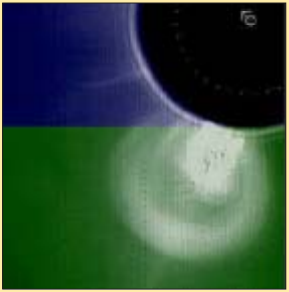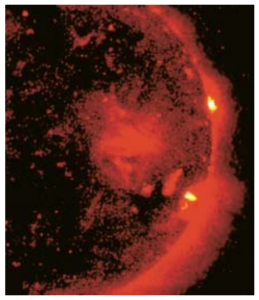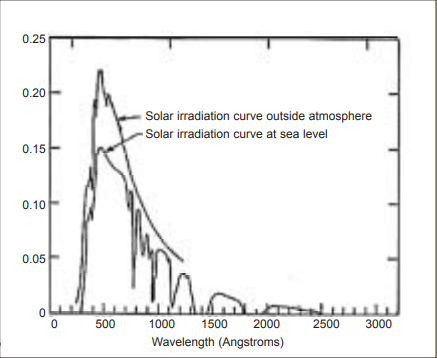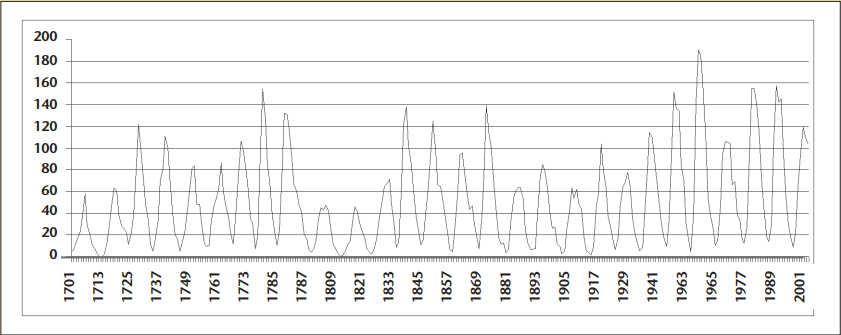Subject:
Mathematics

Topics: RatiosGeometry (Circles)Geometry (surface area)GeometryScientific NotationAlgebraMeasurement (wavelength)ProbabilityMeasurement (general)
Common Core State Standard: 6.RP.1, 7.G.6, 8.EE.4, F-IF.4, S-CP.2, S-CP.7,
Lesson:

Background: The sun is the source of the light and heat that sustains virtually all of Earth’s inhabitants; its rise and fall is perhaps the most constant phenomenon of our lives. Yet the sun is also a place of continuous,  unimaginably violent explosions of matter and energy, and even 93 million miles away we would not long survive its direct energy without the protection of our atmosphere and the Earth’s magnetic field.

In fact, what we think of as the empty space of the solar system is filled with charged particles streaming outward from the sun at a million miles an hour. And from time to time, when its surface emits terrific outbursts called “coronal mass ejections”, the sun flings outward a huge pulse of extremely high energy particles and radiation, powerful enough to warp the Earth’s magnetic field, disrupt the operation of communications satellites, and threaten the health of astronauts.

In an era when a significant amount of humankind’s activities take place outside the atmosphere, the ability to detect—and predict—such phenomena is vital to commerce and space exploration.

Curriculum Connections:

Ratios, Measurement, Geometry (circles)This image shows the sun (black disk) ejecting a large ball of roughly spherical material. Measure the radius of the sun and the radius of the ejected material, and write a ratio that compares them.

Probability

This chart shows some of the information of the National Oceanographic and Atmospheric Administrations “Space Weather” scale, which describes the severity of solar radiation events.

The last column describes how frequently each type of event might be expected over an 11-year period.

a) What is the probability that at least one of these events will occur on any given day?

b) What is the probability that a potentially hazardous event might occur at some point during a 10-day space mission?

 Category Descriptor Effect Average Frequency S5 Extreme Unavoidable high radiation hazard to astronauts on EVA (extra-vehicular activity); high radiation exposure to passengers and crew in commercial jets at high latitudes (approximately 100 chest x-rays) is possible. Fewer than 1 day per 11 years S4 Severe Unavoidable radiation hazard to astronauts on EVA; elevated radiation exposure to passengers and crew in commercial jets at high latitudes (approximately 10 chest x-rays) is possible. 3 days per 11 years S3 Strong Radiation hazard avoidance recommended for astronauts on EVA; passengers and crew in commercial jets at high latitudes may receive low-level radiation exposure (approximately 1 chest x-ray). 10 days per 11 years S2 Moderate No hazard 25 days per 11 years S1 Minor No hazard 50 days per 11 years

Geometry (surface area), Scientific NotationThe term “solar constant” refers to the average amount of solar energy that reaches the Earth. It is equal to 2 calories per square centimeter of area, every minute.

This energy does not reach only the Earth; it flows outward from the sun in all directions. Imagine a sphere, with its center at the sun and a radius equal to the distance from the sun to the Earth: each square centimeter
of that sphere’s surface receives about 2 calories of energy every minute.

Calculate the total number of calories that strikes the entire area of that imaginary sphere per minute.

Compare this to the annual energy consumption of the United States, which is roughly 2.5 x 1019 calories. For how many years could 1 minute’s total energy output of the sun supply the U.S. with energy?

Measurement (wavelength)

This graph shows the intensity for a range of wavelengths of sunlight above the atmosphere (top curve) and at ocean level on Earth (bottom curve). Study the graph and do research as necessary to answer these questions:

a) Why does the sun appear to be yellow?

b) By approximately what percentage is the most intense wavelength reduced in energy by its passage through the atmosphere?

c) The atmosphere’s ozone layer protects us from certain kinds of ultraviolet radiation. What evidence can you see for that fact in the graph?Algebra (patterns)

This graph shows the variation of sunspot activity over the past 300 years. What patterns do you see?Algebra (solving equations), Scientific Notation

Any particles which fly off the surface of the sun, such as the solar wind and coronal mass ejections, must overcome the pull of the sun’s gravity to do so. How powerful is that pull? You might expect it to be hundreds of thousands of times the pull of Earth’s gravity, since the sun’s mass is about 300,000 times the mass of the Earth. However, the fact that the sun is such a large body also means that its surface is a long way from its center, which reduces the gravitational pull.

On the surface of the Earth, the pull of gravity is strong enough to cause any falling body to accelerate towards the ground at the rate of 9.8 meters per second each second (for each second of falling, the velocity increases by 9.8 meters per second). You can find the gravitational pull on the surface of the sun with this equation:

GMm / R2 = ma

This equation states that the force of gravity at the surface of the sun, which according to Newton’s law of gravitation is given by the expression on the left, is equal to the mass of an object (m) multiplied by the acceleration that is caused by that force.

Solve this equation for a, the acceleration at the surface of the sun, using the fact that G = 6.67 x 10-11 kgm3/second2, the radius of the sun (R) is 7 x 108 km, and the mass of the sun, M, is equal to 2 x 1030 kg. About
how many times stronger than the Earth’s pull is the sun’s pull at its surface?